# Alice Unit 3-4 Assignments

FIRST:  make sure you know:  how to use What as a String and perform Math expressions  . You will not be able to do this assignment until you understand both of the above lessons.

## Assign 1) Area of Triangle

Reference packets : #11, #12

Create a world that has a character (person/penguin/whatever) that will ask the user for the height of a triangle and the base of a triangle, and  calculate its area1. 3  different variables  : height , base  and  area  of the triangle
2.  ask the user for  a number  for the height of the triangle and store it into  height (How to ask user for a number)
3.  ask the user for  a number  for the base  of the triangle and store it into  base(ask user for a number)
4.   use area formula and Alice Math expressions to compute the area.  (Note: if you don’t know how to use Math, refer to packet 11 or read this post  )
6. Have your character say the area of the triangle  (remember  area  is a number. You must use what as a string to say it.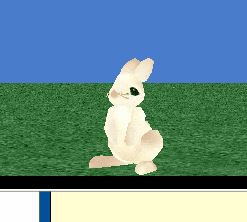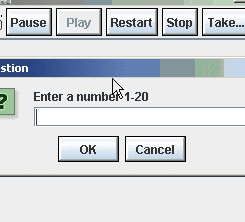## Assign 2) Roman Numeral Translator

Objective : to let the user type in a number between 1 and 10 and then have a character “translate” that into Roman Numerals ( I, II, III, IV, V, VI, VII, VIII, IX, X)

• Ask the user for a number and store the return value into a variable called  numberToTranslate
• use a series of “if/else tests to translate the number and say the appropriate Roman translation for each number between 1 and 10
• Use “==” (link )  to test if two values are the same.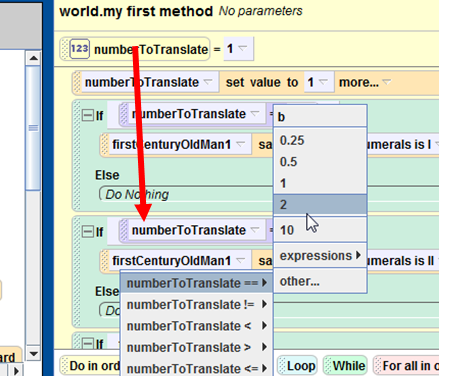• if the user enters a number greater than 10 then  OR less than 1 , have your character say, use either a or b “Only enter numbers between 1 and 10”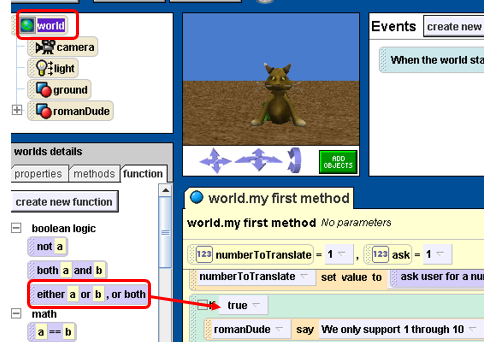Final result :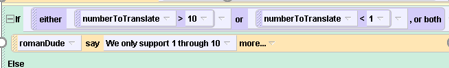We are going to use the roman Numeral Translator file again, so it’s important that you don’t lose the file and give it a good name that you can easily recognize in the future.

Extra credit: Translate 1-20 (Note : You still need to display an error if they enter a number that is too big, which in this case would be >21)

## Assign 3) MPG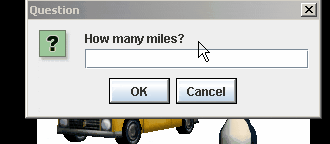Create a world in which the

• At the end , your character should say the value of the mpg  . (You must use what as a string )

Lastly,

• use an if/else  as follows
• if mpg is less  than 30, say “wow, what a gas guzzler”
• else say “This car gets pretty good gas mileage”

## Assign 4) MPG  Temp Converter with if/else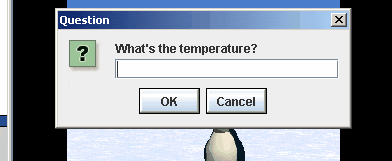• Create a variable called degreesFahrenheit   .
• Store the return value of the ask user for a number function in  degreesFahrenheit
• Create a variable called  degreesCentigrade
• Use the following formula to convert the degreesFahrenheit   to Centigrade using the following formula below:

• use a character from the`People `collection to say the centigrade temperature.
• Don’t forget that you can’t say numbers, you must use what as a string
• use an if/else so that you say “Wow it’s cold” If the final temperature is less than 5 and “Whew it’s hot” if the temperature is greater than 30  (Centigrade)

Assignment 5) Circle’s Area and Circumference: Create a world with your choice of person from the `People`collection. When you play the world, it should

• Ask the user for the radius of a circle. (This value should be stored in a variable ).
• Use a circle shape to represent the circle.
• The person should then say the area and the circumference of the circle.( Links for the circumference formula , area of a circle)
• (For an extra point do the same thing with a “Sphere“. Ask the user for the radius and calculate the surface area and the volume.)  This should be done in the same file as the circle.

# Rock Paper Scissors

Assignment 5-extra credit ). Look up the formulas for the volume and surface area of a cylinder . Ask the user for the height of the cylinder and the radius, which should be stored in variables. Then calculate the surface area and volume of the cylinder . See if there is a cylinder shape in the gallery to use as a visual aid. And have an object say the volume and surface areas.